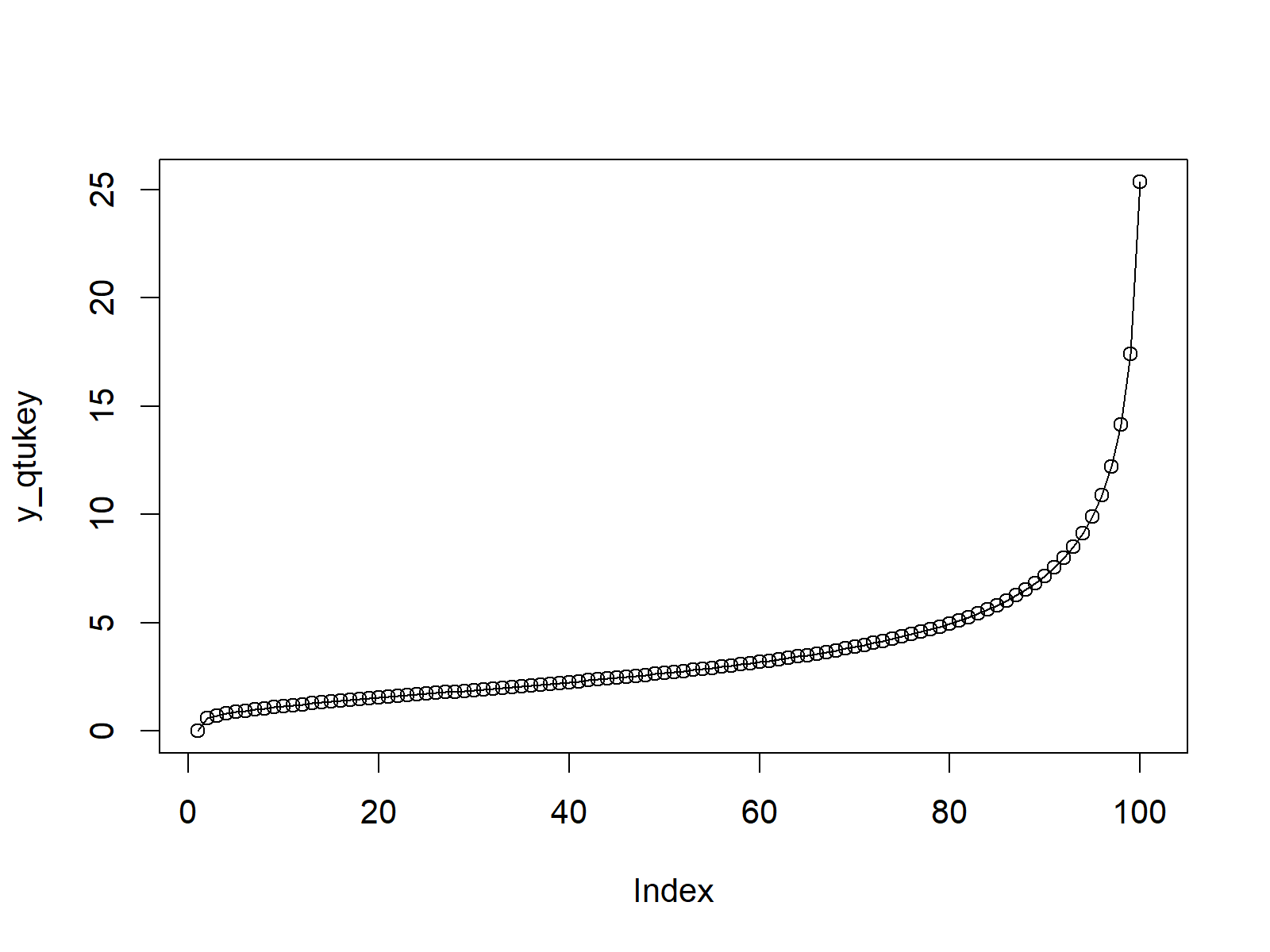# Studentized Range Distribution in R (2 Examples) | ptukey & qtukey Functions

In this tutorial, I’ll explain how to apply the Studentized Range distribution functions in R.

Here’s how to do it.

## Example 1: Studentized Range Cumulative Distribution Function (ptukey Function)

Example 1 shows how to draw a plot of the Studentized Range cumulative distribution function (CDF) in R:

```x_ptukey <- seq(0, 30, by = 1)                       # Specify x-values for ptukey function
y_ptukey <- ptukey(x_ptukey, nmeans = 5, df = 2)     # Apply ptukey function
plot(y_ptukey, type = "o")                           # Plot ptukey values```Figure 1: CDF of Studentized Range Distribution in R.

## Example 2: Studentized Range Quantile Function (qtukey Function)

The second example illustrates how to plot the quantile function of the Studentized Range distribution in R:

```x_qtukey <- seq(0, 1, by = 0.01)                     # Specify x-values for qtukey function
y_qtukey <- qtukey(x_qtukey, nmeans = 5, df = 2)     # Apply qtukey function
plot(y_qtukey, type = "o")                           # Plot qtukey values```Figure 2: Quantile Function of Studentized Range Distribution in R.

## Video & Further Resources

Do you need further explanations on the R codes of this tutorial? Then you might want to have a look at the following video of my YouTube channel. In the video, I show the contents of this article in a live session:

You might also have a look at the other posts on distributions and the simulation of random numbers in the R programming language:

In addition, you may read the related R programming articles of my website.

In summary: This tutorial illustrated how to use the Studentized Range commands in the R programming language. If you have additional questions, don’t hesitate to let me know in the comments section below.

Subscribe to the Statistics Globe Newsletter

•Endalew
July 7, 2021 7:04 am

How to calculate and plot the probability of excedency and cumulative probability for 30 years annual rainfall data?

•July 7, 2021 2:09 pm

Hey Endalew,

For more precise tips, you would have to be more specific about your data.

However, you may have a look at the following tutorials for some hints:

Regards

Joachim